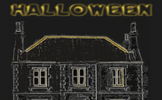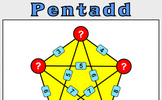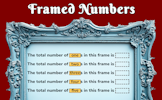# Testing conjectures

### Term 1 starting in week 5 :: Estimated time: 2 weeks

• Factors, Multiples and Primes (review)
• True or False?
• Always, Sometimes, Never true
• Show that
• Expand a pair of binomials
• Conjectures with algebra
• Explore the 100 grid

This page should remember your ticks from one visit to the next for a period of time. It does this by using Local Storage so the information is saved only on the computer you are working on right now.

## Lesson Starters

Here are some suggestions for whole-class, projectable resources which can be used at the beginnings of each lesson in this block.

### 1st Lesson#### Halloween

Five problems with a Halloween theme.

### 2nd Lesson#### Four Factors

Find four single digit numbers that multiply together to give 120. How many different ways are there of answering this question?

### 3rd Lesson#### Summer Holidays

How many children and how many donkeys are on the beach? You can work it out from the number of heads and the number of feet!

### 4th LessonFive numbers are added together in pairs and the sums shown. What might the five numbers be?

### 5th Lesson#### Four Make 999

Find four numbers from those given that add up to 999. How many different sets of four numbers can be found?

### 6th Lesson#### Half a Pound

If A costs 1p, B costs 2p, etc can you find a word which costs exactly 50p?

Some of the Starters above are to reinforce concepts learnt, others are to introduce new ideas while others are on unrelated topics designed for retrieval practice or and opportunity to develop problem-solving skills.

White Rose ResourcesEnd of block assessments provide a quick progress check at the end of each block of learning to make sure students have understood the content covered. This Scheme of Learning was produced by White Rose Maths and is used here with permission granted on 30th June 2021.For All: